# Tutorial on the snha package

#### 2023-03-05

Abstract

The snha package provides easy to use R functions to apply the St. Nicolas House Analysis to your data. The algorithm traces associations chains between interactiving variables. The algorithm was described recently by Groth et. al. (2019)1 and more detailed by Hermanussen et. al. (2021)2. In this package vignette the basic workflow for analyzing your and raw data and as well for analysing precomputed correlation matrices is demonstrated.

## Introduction

The package snha explores interacting variables by searching association chains where correlation coefficients between variables drop in a regular order between a set of variables. The package can be used by calling the function snha with your data, where the columns must be your variables. The return value is an object of class snha which can be visualized using a plot function. The details of the analysis can be inspected by looking at the internal variables of this object. Below follows a minimal analysis for the birthwt data from the MASS R package. The variables are:

• age - mother’s age in years.
• lwt - mother’s weight in pounds at last menstrual period.
• race - mother’s race (1 = white, 2 = black, 3 = other).
• smoke - smoking status during pregnancy (0 = no, 1 = yes).
• ptl - number of previous premature labours.
• ht - history of hypertension (0 = no, 1 = yes).
• ui - presence of uterine irritability (0 = no, 1 = yes).
• ftv - number of physician visits during the first trimester.
• bwt - birth weight of child in grams.

Let’s start with the data preparation. For illustrative purposes we add a random data vector as well:

set.seed(125)
# retrieve the data
library(MASS)
data(birthwt)
birthwt$low=NULL # remove column for the low indicator # which is 1 i a child has low birtwt rnd=round(rnorm(nrow(birthwt),mean=10,sd=2),2) # rnd just contains random data birthwt=cbind(birthwt,rnd=rnd) # adding it head(birthwt) ## age lwt race smoke ptl ht ui ftv bwt rnd ## 85 19 182 2 0 0 0 1 0 2523 11.87 ## 86 33 155 3 0 0 0 0 3 2551 8.95 ## 87 20 105 1 1 0 0 0 1 2557 13.63 ## 88 21 108 1 1 0 0 1 2 2594 10.17 ## 89 18 107 1 1 0 0 1 0 2600 10.79 ## 91 21 124 3 0 0 0 0 0 2622 5.61 OK, we are ready to go: We added the random data column rnd and removed the redundant column low which indicated low birth weight, for this we have the bwt column in the data set. So we do not need a redundant variable. Let’s now first start for illustrative purposes with a PCA and then with our SNHA method where we use Spearman correlation as it is more robust against outliers than Pearson correlation, we set the p-value threshold, alpha to 0.1 as the algorithm is very resistant against the detection of spurious correlations. opar=par(mfrow=c(1,2),mai=c(0.8,0.8,0.1,0.2)) library(snha) # retrieve some data pca=prcomp(t(scale(birthwt))) summary(pca) ## Importance of components: ## PC1 PC2 PC3 PC4 PC5 PC6 PC7 ## Standard deviation 6.1327 5.4004 5.0449 4.5524 4.4129 4.13882 4.05837 ## Proportion of Variance 0.1972 0.1529 0.1335 0.1087 0.1021 0.08982 0.08636 ## Cumulative Proportion 0.1972 0.3501 0.4836 0.5922 0.6943 0.78416 0.87052 ## PC8 PC9 PC10 ## Standard deviation 3.56093 3.466 1.602e-15 ## Proportion of Variance 0.06649 0.063 0.000e+00 ## Cumulative Proportion 0.93700 1.000 1.000e+00 plot(pca$x[,1:2],xlab='PC1', ylab='PC2',pch=19,cex=5,col='salmon')
text(pca$x[,1:2],colnames(birthwt)) text(-5,-10,"PCA",cex=2) as=snha(birthwt,method="spearman",alpha=0.1) par(mai=c(0.8,0.2,0.1,0.2)) plot(as,layout="sam",vertex.size=7,lwd=3,edge.width=3) text(-1.5,-1.8,"SNHA",cex=2) box()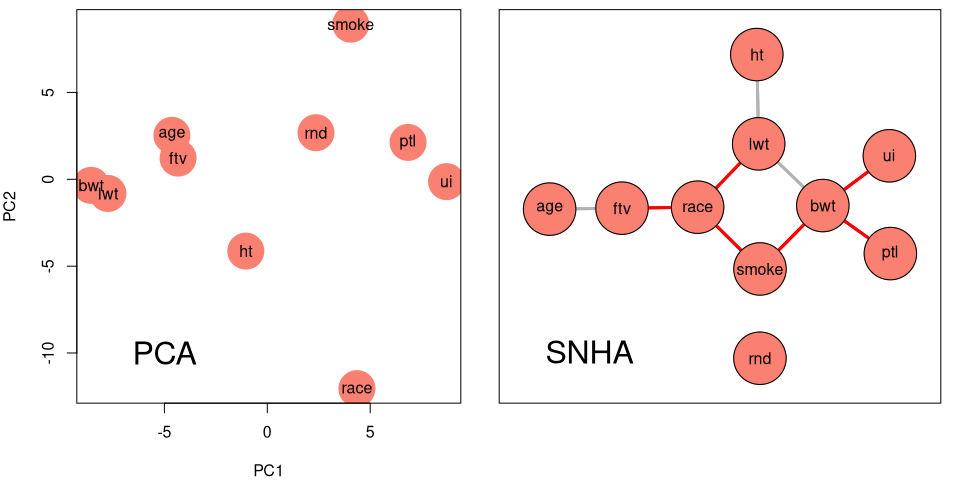Birth weight data variable interactions par(opar) In the PCA plot on the left, the most import variables, having high values in the first component, are on the left and right borders of the plot, unimportant variables are in the center, negatively associated deeply interacting variables such as birthweight (bwt) and premature labours (ptl) are on opposite sides of the plot. These characteristics of the PCA plot make it hart to follow the variable relations. In contrast the variables in the SNHA graph on the right show immediately logical interactions, the birth weight is positively associated to mothers last weight, and negatively to smoking, premature labours and uterine irritability, white people smoke more and white mothers visit more often physicians … The older the mother the more visits at physicians and hypertension is positively associated with weight of the mother. What are the R-square values, the prediction power for every node based on linear models and what are the connections between the variables stored in the adjacency matrix ‘theta’: round(snha_rsquare(as),2) ## age lwt race smoke ptl ht ui ftv bwt rnd ## 0.05 0.12 0.15 0.18 0.02 0.06 0.08 0.05 0.13 0.00 as$theta
##       age lwt race smoke ptl ht ui ftv bwt rnd
## age     0   0    0     0   0  0  0   1   0   0
## lwt     0   0    1     0   0  1  0   0   1   0
## race    0   1    0     1   0  0  0   1   0   0
## smoke   0   0    1     0   0  0  0   0   1   0
## ptl     0   0    0     0   0  0  0   0   1   0
## ht      0   1    0     0   0  0  0   0   0   0
## ui      0   0    0     0   0  0  0   0   1   0
## ftv     1   0    1     0   0  0  0   0   0   0
## bwt     0   1    0     1   1  0  1   0   0   0
## rnd     0   0    0     0   0  0  0   0   0   0

It can be seen that the overall strength of the association is very small, largest r-square value is 0.18 for smoke, 0.13 for birthweight (bwt) but still the analysis show reasonable results without having the necessity of finding some optimal threshold.

## Decathlon data

Here is an other example where we analyze the relationship between the different decathlon disciplines with athletes taking part in the 1988 Olympics and which had results above 7000 points. We perform the St. Nicolas House Analysis and later check the average R-square values for the each node.

### data loading
data(decathlon88)
head(decathlon88)
##    disc high  jave long pole  shot  X100  X110 X1500  X400
## 1 49.28 2.27 61.32 7.43  4.7 15.48 32.00 26.17 20.08 29.45
## 2 44.36 1.97 61.76 7.45  5.1 14.97 33.12 27.39 19.78 30.18
## 3 43.66 1.97 64.16 7.44  5.2 14.20 32.20 26.74 20.52 29.82
## 4 44.80 2.03 64.04 7.38  4.9 15.02 33.90 26.90 18.94 29.35
## 5 41.20 1.97 57.46 7.43  5.2 12.92 32.67 27.50 21.04 30.35
## 6 43.06 2.12 52.18 7.72  4.9 13.58 33.24 27.93 19.70 29.79
A=snha(decathlon88,method="spearman",alpha=0.1)
cols=rep("salmon",10)
cols[names(A$data) %in% c("jave","shot","disc","pole")]="skyblue" plot(A,layout="sam",vertex.color=cols,vertex.size=8,cex=1.1,edge.width=5) snha_rsquare(A) ## disc high jave long pole shot X100 ## 0.68526777 0.09393084 0.35741977 0.36944843 0.40512466 0.75415820 0.52515892 ## X110 X1500 X400 ## 0.53154849 0.43997638 0.56366090 mn=mean(snha_rsquare(A)) title(paste("R-square = ",round(mn,2)))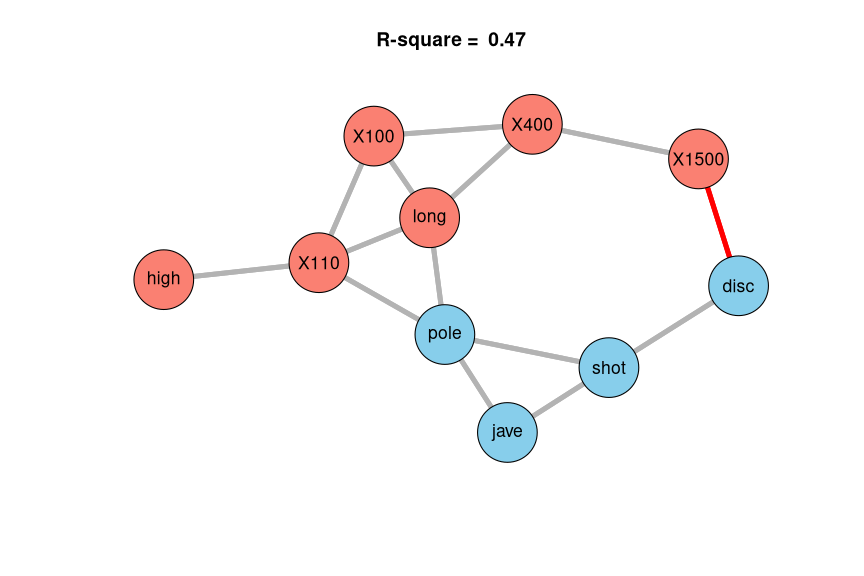SNHA - Decathlon Data 1988 As you can see the variables nicely separates between disciplines related to the upper part of the body (blue) and disciplines where the legs do most of the work (salmon). The mostly hated 1500m run is negatively associated to the throwing disciplines. The running distances are building a chain 100-400-1500m as expected and the jump disciplines are close to each other high-jump (high), hurdles (X110), long jump (long) and pole. As you can see the variables are just in their logical order. round(A$sigma,2)
##        disc high  jave long pole  shot X100 X110 X1500  X400
## disc   1.00 0.05  0.42 0.18 0.37  0.79 0.06 0.16 -0.37 -0.11
## high   0.05 1.00  0.11 0.21 0.32  0.12 0.27 0.42  0.08  0.11
## jave   0.42 0.11  1.00 0.32 0.35  0.64 0.11 0.17  0.00 -0.07
## long   0.18 0.21  0.32 1.00 0.37  0.19 0.55 0.46  0.27  0.41
## pole   0.37 0.32  0.35 0.37 1.00  0.50 0.43 0.55  0.11  0.32
## shot   0.79 0.12  0.64 0.19 0.50  1.00 0.17 0.25 -0.24 -0.11
## X100   0.06 0.27  0.11 0.55 0.43  0.17 1.00 0.67  0.22  0.61
## X110   0.16 0.42  0.17 0.46 0.55  0.25 0.67 1.00  0.12  0.51
## X1500 -0.37 0.08  0.00 0.27 0.11 -0.24 0.22 0.12  1.00  0.54
## X400  -0.11 0.11 -0.07 0.41 0.32 -0.11 0.61 0.51  0.54  1.00
round(A$p.value,3) ## disc high jave long pole shot X100 X110 X1500 X400 ## disc 0.000 0.792 0.016 0.328 0.033 0.000 0.755 0.361 0.034 0.528 ## high 0.792 0.000 0.547 0.242 0.065 0.498 0.130 0.016 0.665 0.551 ## jave 0.016 0.547 0.000 0.074 0.044 0.000 0.540 0.337 0.990 0.719 ## long 0.328 0.242 0.074 0.000 0.033 0.295 0.001 0.007 0.130 0.018 ## pole 0.033 0.065 0.044 0.033 0.000 0.003 0.013 0.001 0.549 0.067 ## shot 0.000 0.498 0.000 0.295 0.003 0.000 0.337 0.160 0.185 0.534 ## X100 0.755 0.130 0.540 0.001 0.013 0.337 0.000 0.000 0.216 0.000 ## X110 0.361 0.016 0.337 0.007 0.001 0.160 0.000 0.000 0.522 0.002 ## X1500 0.034 0.665 0.990 0.130 0.549 0.185 0.216 0.522 0.000 0.001 ## X400 0.528 0.551 0.719 0.018 0.067 0.534 0.000 0.002 0.001 0.000 For illustrative purposes create a graph with the same layout but with edges showing all significant correlations. B = A$theta
B[]=0
B[A$p.value<0.05]=1 diag(B)=0 plot.snha(B,layout='sam',vertex.color=cols,vertex.size=8,cex=1.1,edge.width=5)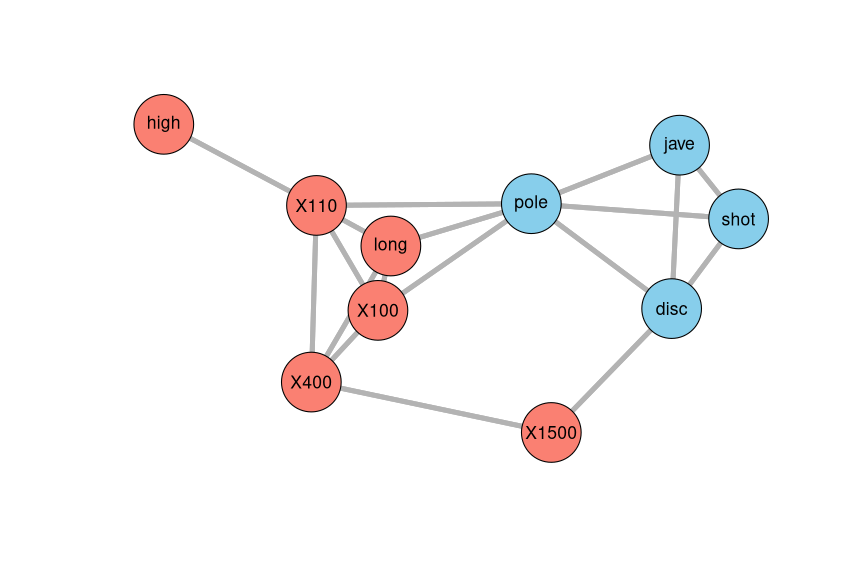Decathlon Data 1988 (p-value Graph) As you can see the major relationships are the same, but there are a few more edges which did however not enhance the overall data structure. In case of really interacting variables it would be as well difficult to distinguish between direct and indirect associations, as the latter can be as well very easily become significant if the primary interaction is highly significant. ## Swiss dataset example Let’s finish with an other data set, the swiss data which are available in every R installation. Here we try out both the correlation methods, Spearman and Pearson correlation. We use the function snha_layout to determine a layout matrix which we will then reuse for both plots. library(snha) data(swiss) head(swiss,4) ## Fertility Agriculture Examination Education Catholic ## Courtelary 80.2 17.0 15 12 9.96 ## Delemont 83.1 45.1 6 9 84.84 ## Franches-Mnt 92.5 39.7 5 5 93.40 ## Moutier 85.8 36.5 12 7 33.77 ## Infant.Mortality ## Courtelary 22.2 ## Delemont 22.2 ## Franches-Mnt 20.2 ## Moutier 20.3 ### shorter names useful for display later in the graph colnames(swiss)=abbreviate(colnames(swiss)) head(swiss,4) ## Frtl Agrc Exmn Edct Cthl In.M ## Courtelary 80.2 17.0 15 12 9.96 22.2 ## Delemont 83.1 45.1 6 9 84.84 22.2 ## Franches-Mnt 92.5 39.7 5 5 93.40 20.2 ## Moutier 85.8 36.5 12 7 33.77 20.3 opar=par(mfrow=c(1,2)) ### options(warn=-1) as=snha(swiss,method="pearson") ### store layout for reuse in two graphs lay = snha_layout(as,mode="sam") plot(as,layout=lay,vertex.size=8,main="Pearson") as=snha(swiss,method="spearman") plot(as,layout=lay,vertex.size=8,main="Spearman")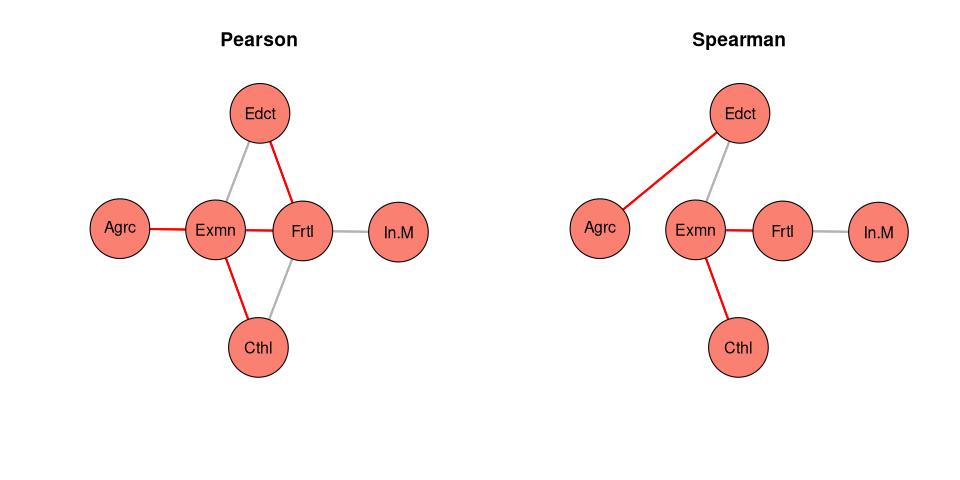Swiss data variable associations par(opar) Here is the resulting adjacency matrix: knitr::kable(as$theta)
Frtl Agrc Exmn Edct Cthl In.M
Frtl 0 0 1 0 0 1
Agrc 0 0 0 1 0 0
Exmn 1 0 0 1 1 0
Edct 0 1 1 0 0 0
Cthl 0 0 1 0 0 0
In.M 1 0 0 0 0 0

As you can see the structure remains the same, but Pearson correlation shows more edges, we should check if the data are normally distributed. Again, without playing around with some parameters or thresholds we get immediately the general associations between the data. Let’s just check if the data are normally distributed and then conclude if we should use Spearman correlation for non-normally distributed data or Pearson correlation for normally distributed data:

### prepare a test returning only p-values
mtest = function (x) { return(shapiro.test(x)$p.value) } df=data.frame(orig=round(apply(swiss,2,mtest),3)) df=cbind(df,log2=round(apply(log2(swiss),2,mtest),3)) knitr::kable(df) orig log2 Frtl 0.345 0.003 Agrc 0.193 0.000 Exmn 0.256 0.006 Edct 0.000 0.257 Cthl 0.000 0.000 In.M 0.498 0.008 As you can see, both with the original data and as well with the log-normalized data the Shapiro-Wilk test has a few significant entries, so we reject the Null-hypothesis that these data are coming from a normal distribution. So for our example using the swiss data we should very likely prefer using the Spearman correlation. ## Plotting The plotting of the graph can be changed in various ways, for details see ?plot.snha. Here I just give a few examples. As the graph is generated based on the underlying pairwise correlations, it might be useful to display the pairwise correlation either in a correlation plot or by adding the correlations values on the edges of the graph. Here an example where we do first a correlation plot and then a plot of the SNHA graph overlaying the edges with the correlation values. opar=par(mfrow=c(1,2),mai=rep(0.2,4)) sw=snha(swiss,method="spearman",alpha=0.1) plot(sw,type="corrplot") plot(as,edge.text=round(as$sigma,2),edge.pch=15,layout='sam')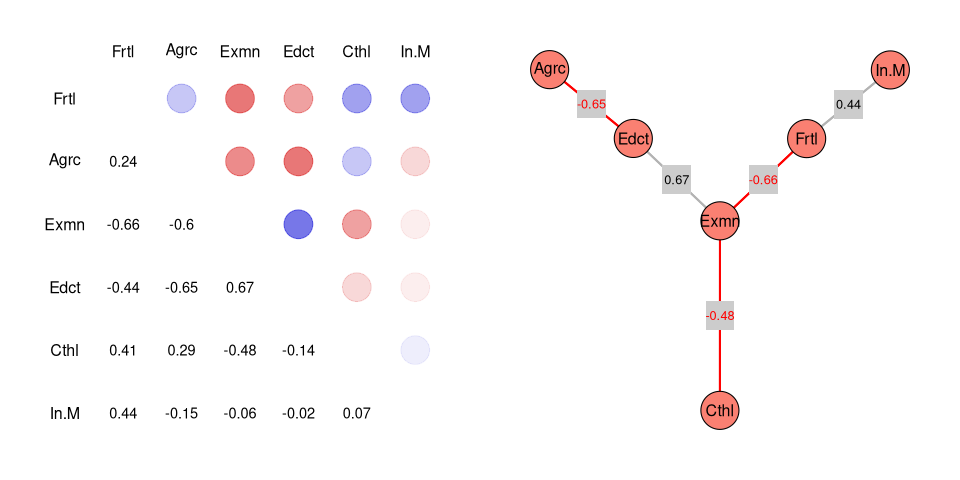Correlation and Network plot with correlation values on the edges

par(opar)

## Log-Likelihood

The edge quality can be judged either by the log-likelihood ratio for the individual chains or by bootstrapping where we look how often a certain chain was found if we do re-samplings with our data set.

Let’s first calculate the log-likelihoods for the different chains which were found. We can see the underlying chains either directly using the internal object chains or by using the snha_get_chains method which returns a data frame:

snha_get_chains(as)
##      Name           Node1  Node2  Node3  Node4
## [1,] "m-chain-Edct" "Agrc" "Edct" "Exmn" "Frtl"
## [2,] "a-chain-Cthl" "Cthl" "Exmn" "Frtl" ""
## [3,] "a-chain-In.M" "In.M" "Frtl" "Agrc" ""

The m in front of a chain name indicated that the chain was found by investigating the variable to be in the middle of a chain, the a indicated that the chain was at the beginning of the investigated chain. For details on the algorithm have a look at Hermanussen et. al. (20212).

The log-likelihood for these chains can be calculated using the function snha_ll like this:

snha_ll(as)
##          chain             members r2sum   r2per  ll.total  ll.chain   ll.rest
## 1 m-chain-Edct Agrc-Edct-Exmn-Frtl 1.314 -116.46 -318.3388 -223.9931 -131.6451
## 2 a-chain-Cthl      Cthl-Exmn-Frtl 0.745  -66.05 -318.3388 -176.5415 -185.3486
## 3 a-chain-In.M      In.M-Frtl-Agrc 0.298  -26.43 -318.3388 -190.9628 -168.9559
##    ll.block df    chisq      p.value block.df  block.ch block.p.value
## 1 -212.5995  7 51.81166 6.359431e-09        3 22.787152  4.472558e-05
## 2 -176.0080  6 86.03570 2.013898e-16        1  1.067003  3.016233e-01
## 3 -189.5145  6 80.26339 3.152138e-15        1  2.896428  8.877610e-02

The relevant p-values are in the last column, if the p-value is higher than 0.05 we can assume that the chain is sufficient to capture the dependency between the variables of the chain. Here for the chain 2 and 3 this is the case, whereas for the first chain the p-value is very low indicating that the chain is not sufficient to capture the variable dependencies. One reason might be that we used Spearman correlation to create the graph whereas log-likelihood assumes linear dependencies.

## Bootstrapping

Another approach to evaluate the quality of chains and edges is bootstrapping. We sample several times items from the data set with replacement and we redo thereafter the analysis with each of the samples. Edges which appear only very rarely are less likely to be of importance and significance.

Let’s use an example:

opar=par(mfrow=c(1,2),mai=c(0.1,0.1,0.7,0.1))
as.boot=snha(swiss,method="spearman",prob=TRUE)
lay=snha_layout(as.boot,method="sam")
plot(as,layout=lay,vertex.size=6,main="Single Run")
plot(as.boot,layout=lay,vertex.size=6,main="Bootstrap Run")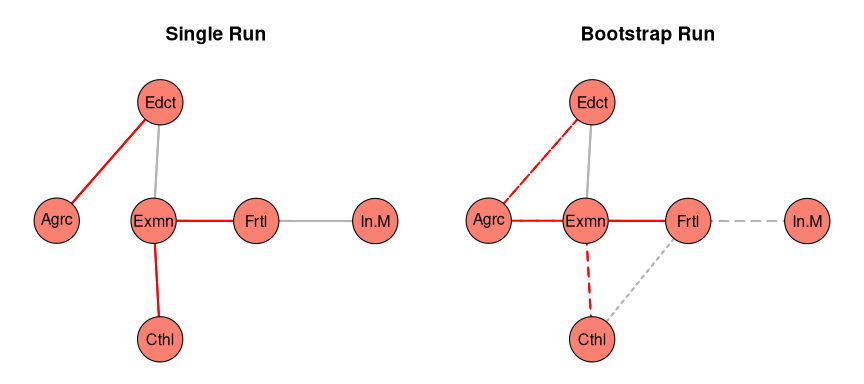Boostrap Example

par(opar)

Solid lines shown in the graph above indicate that edges where found in more than 75 percent of all re-samplings, broken lines indicate edges appearing in more than 50% of all re-samplings and dotted lines in 25-50% of all re-samplings.

As you can see the bootstrap method does find a few more edges than the single run variation of the snha method. If you network is not too large it is usually recommended to use bootstrapping to get more insights into the edge quality and to get as well edges if the network is more dense and has a lot of highly connected nodes.

In order to test the algorithm there is as well in the package a function which allows you to generate data for directed and undirected graphs, either using the given adjacency matrix as precision matrix or using a Monte Carlo simulation as described by Novine et. al (20213). Here an example:

W=matrix(0,nrow=6,ncol=6,dimnames=list(LETTERS[1:6],LETTERS[1:6]))
W[1:2,3]=1
W[3,4]=1
W[4,5:6]=1
W[5,6]=1
W
##   A B C D E F
## A 0 0 1 0 0 0
## B 0 0 1 0 0 0
## C 0 0 0 1 0 0
## D 0 0 0 0 1 1
## E 0 0 0 0 0 1
## F 0 0 0 0 0 0

For such an adjacency matrix we can create data like this:

data=snha_graph2data(W)
dim(data)
##    6 100
round(cor(t(data)),2)
##      A     B    C    D     E    F
## A 1.00  0.10 0.54 0.16  0.13 0.14
## B 0.10  1.00 0.50 0.29 -0.04 0.18
## C 0.54  0.50 1.00 0.31  0.08 0.13
## D 0.16  0.29 0.31 1.00  0.27 0.39
## E 0.13 -0.04 0.08 0.27  1.00 0.48
## F 0.14  0.18 0.13 0.39  0.48 1.00

As you can see the correlations follow the given graph, we can as well plot these for better illustration:

opar=par(mfrow=c(1,3),mai=rep(0.2,4))
plot.snha(W)
plot.snha(cor(t(data)),type="cor")
plot.snha(snha(t(data)))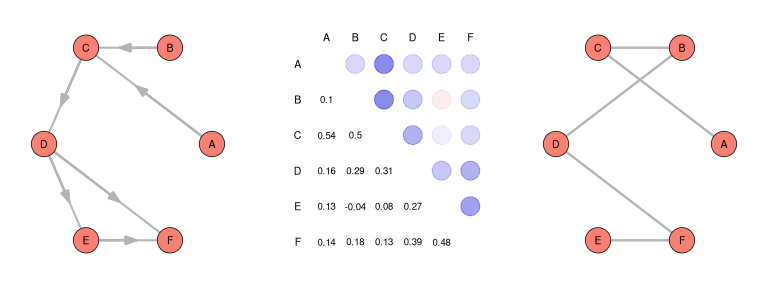True graph, correlations and predicted graph (left to right)

par(opar)

## Installation

As long as the package is not yet on the CRAN repository the package can be usually installed using the submitted tar.gz archive with the following commands:

library(tcltk)
pkgname=tclvalue(tkgetOpenFile(
filetypes="{{Tar.gz files} {*.tar.gz}} {{All files} {*.*}}"))
if (pkgname != "") {
install.packages(pkgname,repos=NULL)
}

It is as well possible to install the latest version directly from the Github repository like this:

library(remotes)
remotes::install_github("https://github.com/mittelmark/snha")


Thereafter you can check the installation like this:

library(snha)
citation("snha")

## Background Details and Concept

Analyzing multivariate data is often done using visualization of pairwise correlations, using principal component analysis or multidimensional scaling as typical methods in this area. The snha package provides an alternative approach, by uncovering ordered sequences of correlation coefficients which can be reversed1 2. Existing chains are translated into edges between the variables, here taken as nodes of a graph. The graph can be then visualized and the major relations between the variables are visible.

The basic assumption of the method is the assumption that correlations coefficients between two variables, where one variable directly influences the other, are larger than those of secondary associations. So for instance if we assume that a variable A influences a variable B, and B influences C, it can be assumed, that r(AB) > r(AC) and that in the opposite direction r(CB) > r(CA).

The algorithm provided in the snha package uncovers such association chains where the order of correlation coefficient can be reversed. The advantage of the method is that there is only a very limited requirement for choosing thresholds for instance for the p-value or for the correlation coefficient. The reason is that the existence of such association chains with the correct ordering of three or more nodes is much less likely to exists by accident then significant pairwise correlations.

In the following we will first illustrate the concept on a simple hypothetical association chain and thereafter you might again study the real world examples at the beginning of this vignette with more understanding.

## Simple association chain

Let’s assume we have a simple association chain where a variable A is influencing a variable B, B is influencing a variable C and C is influencing variable D like this:

opar=par(mai=c(0.1,0.1,0.1,0.0))
plot(1,xlab="",ylab="",axes=FALSE,type="n",xlim=c(0.5,4.5),ylim=c(0.8,1.2))
arrows(1:3,rep(1,3),1:3+0.8,rep(1,3),lwd=3,length=0.1)
points(1:4,rep(1,4),pch=19,col="salmon",cex=6)
text(1:4,1,LETTERS[1:4],cex=2)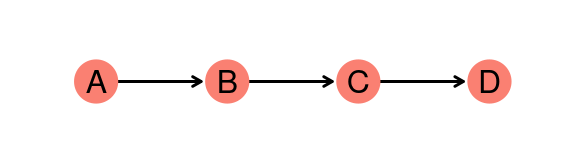An association chain

par(opar)

In this situation we can assume that, despite of the omnipresent noise in such situation, the correlations of directly interacting variables is higher in comparison to variables only connected only via other variables. Let’s assume for simplicity reasons, that the correlation between directly connected variables drops down from r=0.7 to around r=0.5 for secondary connected variables and r=0.3 for tertiary connected variables. So a possible correlation matrix could look like this:

C=matrix(c(1,0.7,0.5,0.3,
0.7,1,0.7,0.5,
0.5,0.7,1,0.7,
0.3,0.5,0.7,1),
nrow=4,byrow=TRUE)
rownames(C)=colnames(C)=LETTERS[1:4]
knitr::kable(C)
A B C D
A 1.0 0.7 0.5 0.3
B 0.7 1.0 0.7 0.5
C 0.5 0.7 1.0 0.7
D 0.3 0.5 0.7 1.0

Let’s now add a little bit of noise and visualize the pairwise correlations using the plot function of the snha package.

set.seed(123)
opar=par(mfrow=c(1,2),mai=c(0.1,0.1,0.1,0.1))
C=C+rnorm(length(C),mean=0,sd=0.1)
C[lower.tri(C)]=t(C)[lower.tri(C)]
diag(C)=1
as=snha(C)
round(as$sigma,3) ## A B C D ## A 1.000 0.713 0.431 0.340 ## B 0.713 1.000 0.655 0.511 ## C 0.431 0.655 1.000 0.644 ## D 0.340 0.511 0.644 1.000 plot(as,type="corplot") as$theta
##   A B C D
## A 0 1 0 0
## B 1 0 1 0
## C 0 1 0 1
## D 0 0 1 0
plot(as)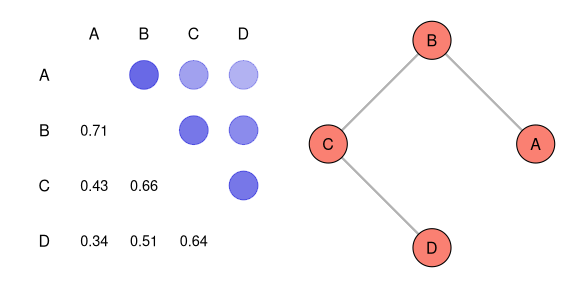Visualization of correlation matrix sigma and the adjacency matrix theta

par(opar)

As we can see, the correlations are now slightly altered. A simple r threshold mechanism, for instance taking only correlations larger than 0.5 into consideration would as well have false positive edges like between the nodes B and D. The function snha takes as input either a correlation matrix or a data matrix or data.frame and tries to find such association chains. The association chain is stored in the internal object theta and can be visualized using the default plot command.

## Summary

Here are the functions to be used by the normal user of the package:

• snha - create a snha graph object
• plot - plot a snha graph object
• as.list - create a list out of a snha graph object, ready to write for instance into an Excel file
• snha_get_chains - get the actual chains which were found and which build the graph
• snha_graph2data - generate for a given adjacency matrix some data
• snha_rsquare - get r-square values for the nodes based on linear model to have a qualitative measure for the graph prediction.

The snha graph object contains a few internal variables which might be of interest for the user:

• alpha - the chosen p-value threshold
• chains - the found association chains
• data - the input data
• method - the correlation method
• p-values the pairwise p-values
• probabilities - in case of bootstrapping the proportion how often a chain was found
• theta - the adjacency matrix for the nodes / variables

## Build information

The package was build using R version 4.1.3 (2022-03-10) on x86_64-redhat-linux-gnu using snha package 0.1.3.

print(sessionInfo())
## R version 4.1.3 (2022-03-10)
## Platform: x86_64-redhat-linux-gnu (64-bit)
## Running under: Fedora Linux 36 (Workstation Edition)
##
## Matrix products: default
## BLAS/LAPACK: /usr/lib64/libflexiblas.so.3.3
##
## locale:
##   LC_CTYPE=en_US.UTF-8       LC_NUMERIC=C
##   LC_TIME=en_US.UTF-8        LC_COLLATE=C
##   LC_MONETARY=en_US.UTF-8    LC_MESSAGES=en_US.UTF-8
##   LC_PAPER=en_US.UTF-8       LC_NAME=C
##  LC_MEASUREMENT=en_US.UTF-8 LC_IDENTIFICATION=C
##
## attached base packages:
##  stats     graphics  grDevices utils     datasets  methods   base
##
## other attached packages:
##  snha_0.1.3  MASS_7.3-55
##
## loaded via a namespace (and not attached):
##   digest_0.6.27   R6_2.5.1        jsonlite_1.7.2  evaluate_0.20
##   highr_0.9       rlang_1.0.2     cachem_1.0.5    cli_3.3.0
##   jquerylib_0.1.4 bslib_0.4.2     rmarkdown_2.20  tools_4.1.3
##  xfun_0.37       yaml_2.2.1      fastmap_1.1.0   compiler_4.1.3
##  htmltools_0.5.4 knitr_1.42      sass_0.4.5